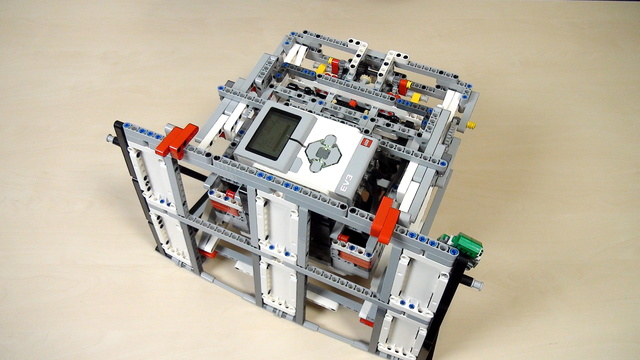В момента ресурсът е наличен само на английски

# Improving FLL Robot Game. Teacher's Note. Correct solution on calculating gears. ProPreview

This is a teacher's note about the math behind calculating gear ratios with for our lifting attachment. It math model we build in previous tutorials is not exactly correct and here is the explanation why.

• #474
• 11 Apr 2017
• 2:17

### Math model calculation

The calculation from the math model shows that 18.75 rotations should be enough to rotate the beam to 90 degrees. What would actually happen is that the driven gear wheel would rotate to 90 degrees and not the beam to which this gear wheel is attached.

### Wrong math model

What we've missed is an important part of the math model. It is a little bit more complicated for the current course and we would explain it in some of the next tutorials. It is a good practice to give this as a task for the students to find our what is wrong with the calculation and the math. They could come ask us. It is a good exercise for every student to try to formulate a question and direct it to us and we would lead them to the answer, or if we've recorded the tutorial we would direct them to the explanation.

### Robot Lifting Attachment With a scissors mechanism

For the FIRST LEGO League 2016-2017 Animal Allies we prepared an attachment for lifting the robot. The idea of the attachment is to show how you could lift the whole robot with a system of gear wheels, levers, and scissors constructions.

Built mainly from LEGO Mindstorms EV3 parts but could probably be constructed from NXT sets.

### „Box“ робот 2 за състезания ЛЕГО Mindstorms

Инструкции за построяването на състезателен робот на принципа на “Box” робота. Този робот е построен с ЛЕГО Mindstorms EV3 и може да се използва на състезания като FLL и WRO.

### English

A short teacher note on the wrong mathematical model here and what's actually the problem there and what are the different losses in the system and how they affect the behavior of our attachment. Our goal was to calculate the number of times that we should rotate this driving wheel in order to have this beam right here rotate to 90 degrees. So, that's the initial position and it should be something like this as a final position. This will make the whole robot move forward and up. And attach. The question to the students was: How many rotations should we make to have this wheel rotate to 90 degrees? And they will find out this. And what they'll notice is if they use 18.75 as we found in the previous video, this gear wheel will rotate to 90 degrees but this will not make the beam rotate to 90 degrees. And because the reason for this and the mathematics is one more level more complex we decided to leave this for the next step. So, we can simulate an engineering process where you do part of the calculation then you experiment with these calculations then you see that your calculations might be wrong because it will be like two times less than the real value. And when you see such a difference between the expected value and the calculated value you should return and do the calculations again. And what we have missed is actually a very important part of the calculation. Again, when rotating with this wheel to 18.75 the final wheel - the driven gear wheel will rotate to 90 degrees. So, 10 teeth but this will not result at the beam rotating to 90 degrees.

## Курсове и занятия включващи този Урок

Този Урок е използван в следните курсове и занятия.• 48
• 118:45
• 15• 8
• 0
• 2
• 3d_rotation 1